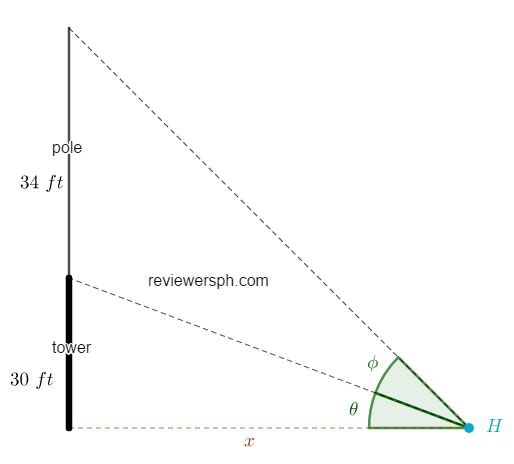### Math Notes

Subjects

#### Trigonometry Solutions

##### Topics || Problems

A flagpole is 34 feet high stands on top of a tower 30 feet high. From a certain point in the same horizontal plane with the base of the tower, the angle subtended by the pole is equal to the angle of elevation of the top of the tower. Find the distance from this point to the base of the tower.

Let $$x$$ be the distance between the base of the tower and the point.

The angle subtended by the pole is $$\phi$$ and the angle of elevation of the top of the tower is $$\theta$$. But these two angles are equal, $$\phi = \theta$$.$$\tan{\theta} = \frac{30}{x}$$

$$\tan{2\theta} = \frac{30+34}{x}$$: since $$\theta = \phi$$

$$\tan{2\theta} = \frac{2\tan{\theta}}{1-(\tan{\theta})^2}$$

$$\frac{64}{x} = \frac{2\frac{30}{x}}{1-(\frac{30}{x})^2}$$

$$\frac{64}{x} = \frac{\frac{60}{x}}{1-\frac{900}{x^2}}$$

$$64 = \frac{60x^2}{x^2-900}$$

$$64(x^2-900) = 60x^2$$

$$64x^2-57600 = 60x^2$$

$$4x^2 = 57600$$

A flagpole is 34 feet high stands on top of a tower 30 feet high. From a certain point in the same horizontal plane with the base of the tower, the angle subtended by the pole is equal to the angle of elevation of the top of the tower. Find the distance from this point to the base of the tower.

Let $$x$$ be the distance between the base of the tower and the point.

The angle subtended by the pole is $$\phi$$ and the angle of elevation of the top of the tower is $$\theta$$. But these two angles are equal, $$\phi = \theta$$.$$\tan{\theta} = \frac{30}{x}$$

$$\tan{2\theta} = \frac{30+34}{x}$$: since $$\theta = \phi$$

$$\tan{2\theta} = \frac{2\tan{\theta}}{1-(\tan{\theta})^2}$$

$$\frac{64}{x} = \frac{2\frac{30}{x}}{1-(\frac{30}{x})^2}$$

$$\frac{64}{x} = \frac{\frac{60}{x}}{1-\frac{900}{x^2}}$$

$$64 = \frac{60x^2}{x^2-900}$$

$$64(x^2-900) = 60x^2$$

$$64x^2-57600 = 60x^2$$

$$4x^2 = 57600$$